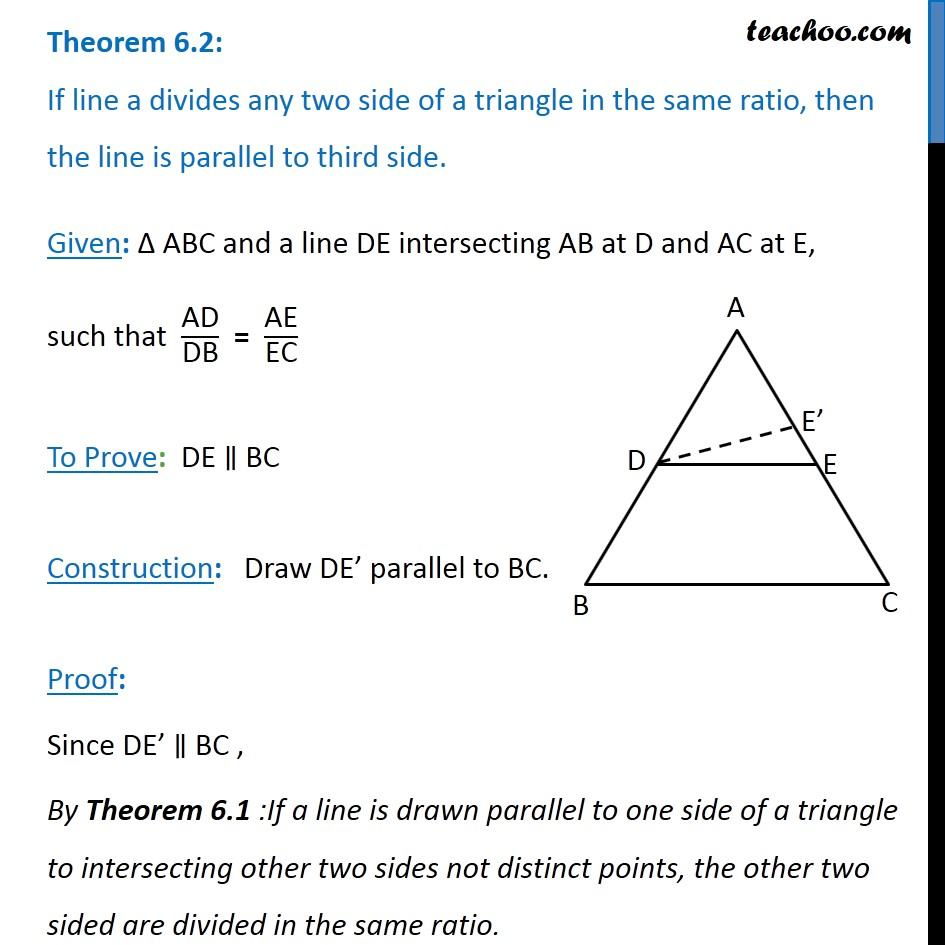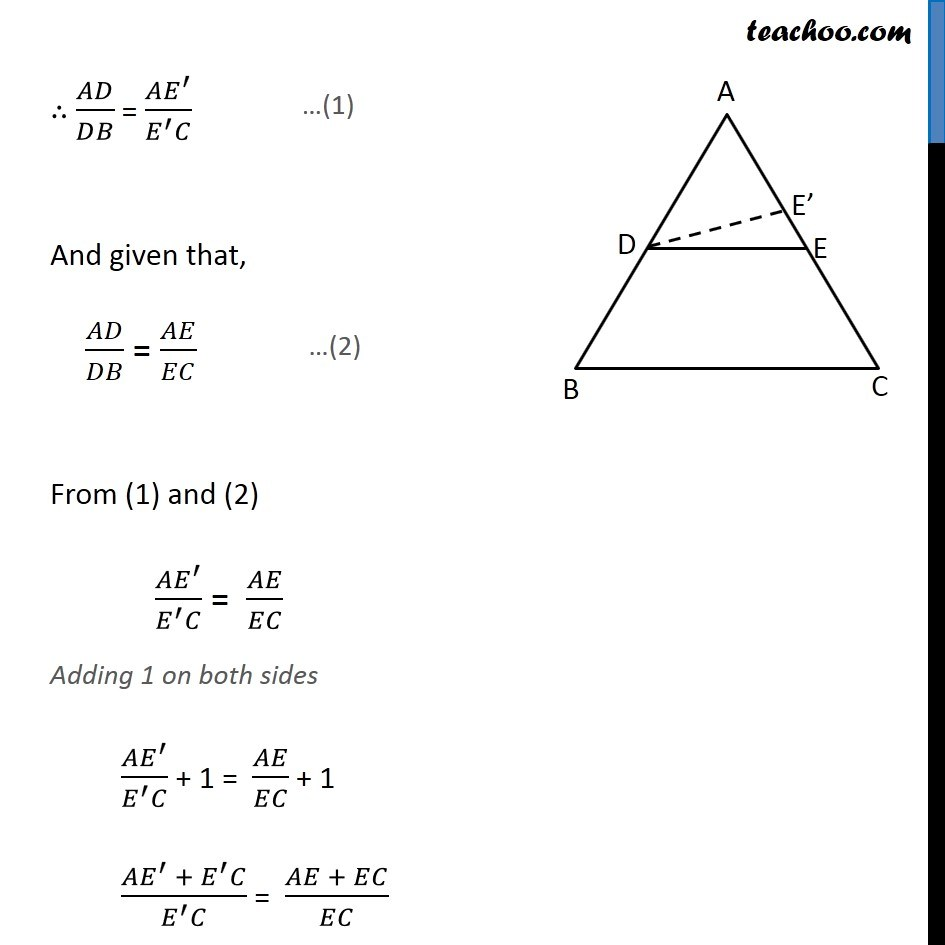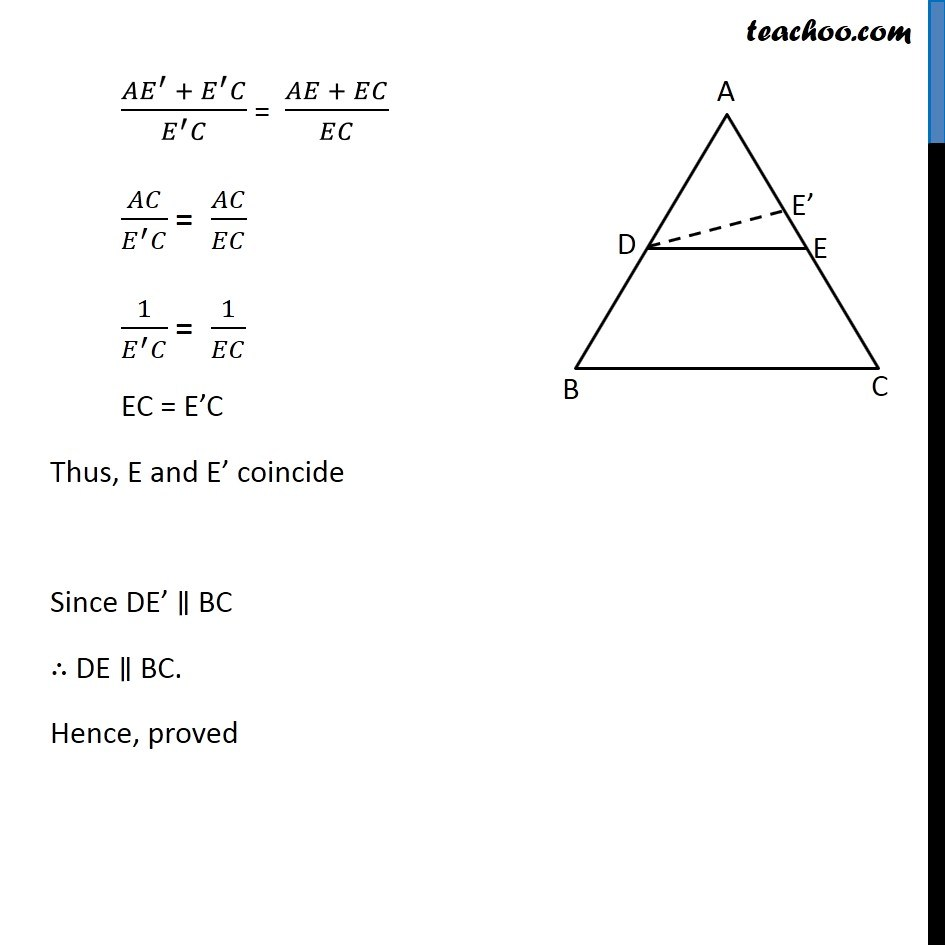Theorem 6.2

Chapter 6 Class 10 Triangles
Concept wiseLearn in your speed, with individual attention - Teachoo Maths 1-on-1 Class

### Transcript

Now, ∆BDE and ∆DEC are on the same base DE and between the same parallel lines BC and DE. ∴ ar (BDE) = ar (DEC) Hence, "ar (ADE)" /"ar (BDE)" = "ar (ADE)" /"ar (DEC)" "AD" /"DB" = "AE" /"EC" Hence Proved. Given: Δ ABC and a line DE intersecting AB at D and AC at E, such that "AD" /"DB" = "AE" /"EC" To Prove: DE ∥ BC Construction: Draw DE’ parallel to BC. Proof: Since DE’ ∥ BC , By Theorem 6.1 :If a line is drawn parallel to one side of a triangle to intersecting other two sides not distinct points, the other two sided are divided in the same ratio. ∴ 𝐴𝐷/𝐷𝐵 = (𝐴𝐸^′)/(𝐸^′ 𝐶) And given that, 𝐴𝐷/𝐷𝐵 = 𝐴𝐸/𝐸𝐶 From (1) and (2) (𝐴𝐸^′)/(𝐸^′ 𝐶) = 𝐴𝐸/𝐸𝐶 Adding 1 on both sides (𝐴𝐸^′)/(𝐸^′ 𝐶) + 1 = 𝐴𝐸/𝐸𝐶 + 1 (𝐴𝐸^′ + 𝐸^′ 𝐶)/(𝐸^′ 𝐶) = (𝐴𝐸 + 𝐸𝐶)/𝐸𝐶 "AE" /"EC" + 1 = "AE′" /"E′C" + 1 ("AE" + "EC" )/"EC" = ("AE′" + "E′C" )/"E′C" "AC" /"EC" = "AC" /"E′C" EC = E’C Thus, E and E’ coincides. Hence, DE ∥ BC. (𝐴𝐸^′ + 𝐸^′ 𝐶)/(𝐸^′ 𝐶) = (𝐴𝐸 + 𝐸𝐶)/𝐸𝐶 𝐴𝐶/(𝐸^′ 𝐶) = 𝐴𝐶/𝐸𝐶 1/(𝐸^′ 𝐶) = 1/𝐸𝐶 EC = E’C Thus, E and E’ coincide Since DE’ ∥ BC ∴ DE ∥ BC. Hence, proved